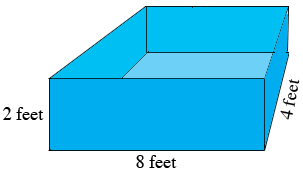SEARCH HOMEMath Central Quandaries & QueriesQuestion from Kareena: I have a rectangular prism shaped pond that are 4ft by 8ft by 2ft. My pond is lined on the sides and bottom with a pool liner so that it doesn't leak (the top of the pool does NOT have a liner). I am redoing my pool and would like to increase the size of the pond. Each dimension will increase by the same amount, and the size of the pool liner will double. How much I am increasing each dimension of the pond? Give an exact answer and than an approx. answer rounded to the nearest tenth.Hi Kareena,

You didn't say so but I am assuming the pond is 2 feet deep. In this case you have two sides 4 feet by 2 feet, two sides that are 8 feet by 2 feet and the bottom which is 4 feet by 8 feet. Hence the area of the existing pool liner is

$2(4 \times 2) + 2(8 \times 2) + 4 \times 8 \mbox{ square feet.}$Suppose each dimension is increased by $x$ feet. Write an expression for the area required for the new liner. This is equal to twice the existing liner.

Solve for $x.$

I hope this helps,
PennyMath Central is supported by the University of Regina and The Pacific Institute for the Mathematical Sciences.